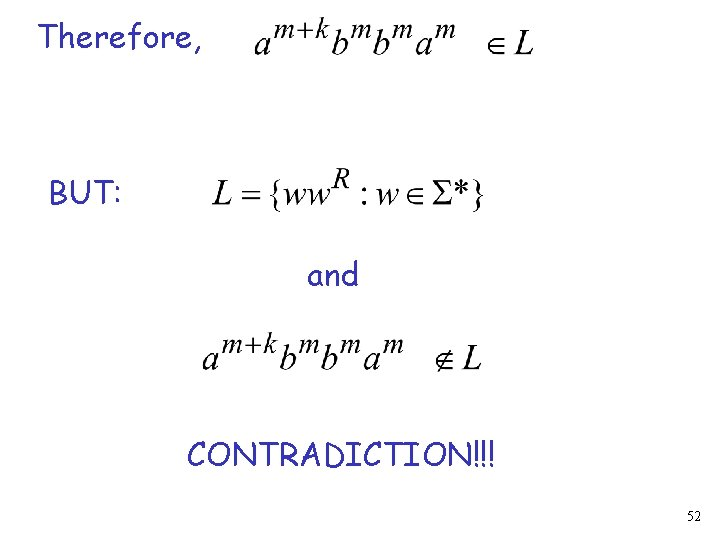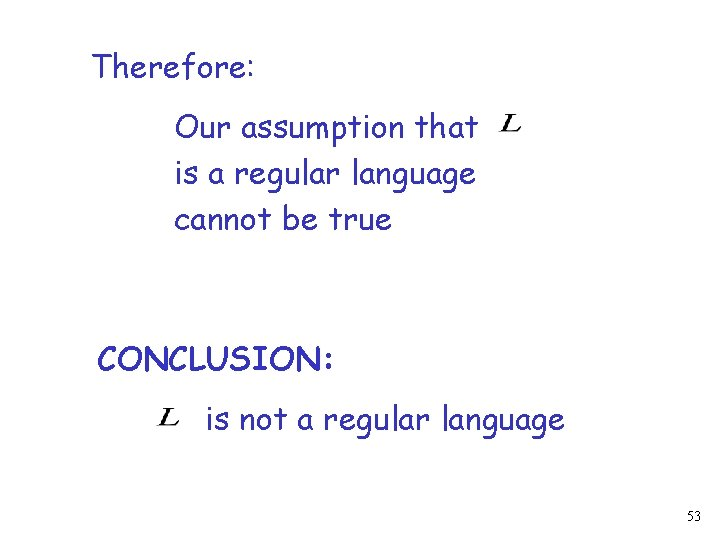# Lets Recapitulate 1 Regular Languages DFAs NFAs Regular

• Slides: 53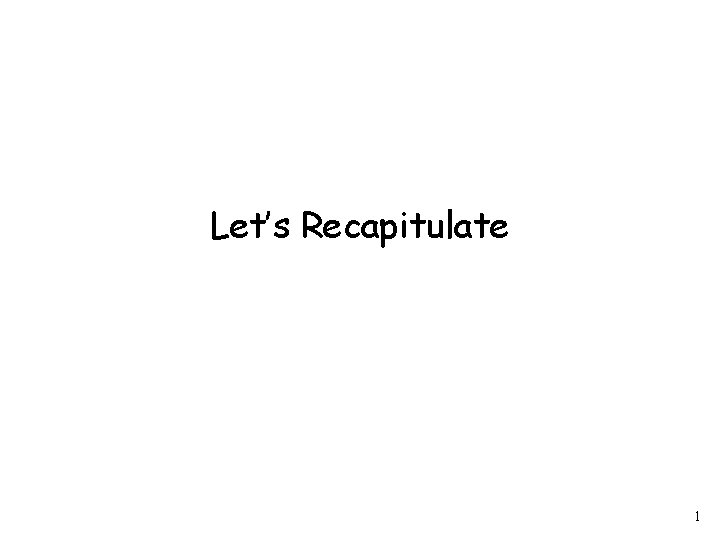Let’s Recapitulate 1Regular Languages DFAs NFAs Regular Grammars Regular Expressions 2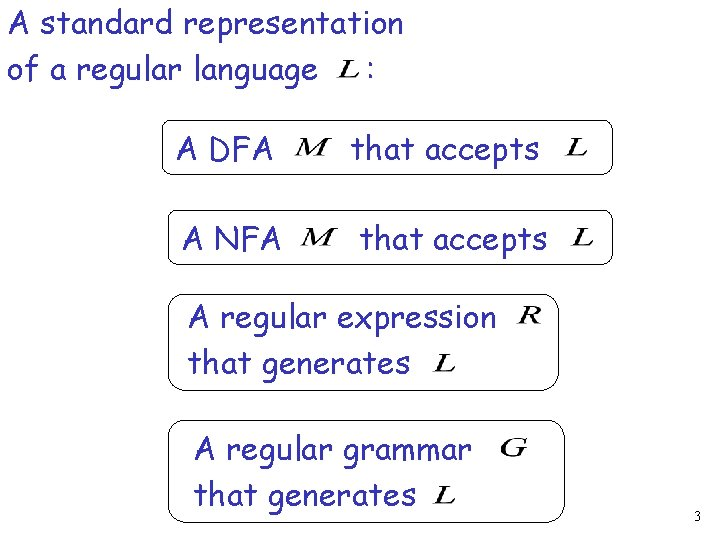A standard representation of a regular language : A DFA that accepts A NFA that accepts A regular expression that generates A regular grammar that generates 3When we say: “We are given a Regular Language “ We mean: Language in a standard representation 4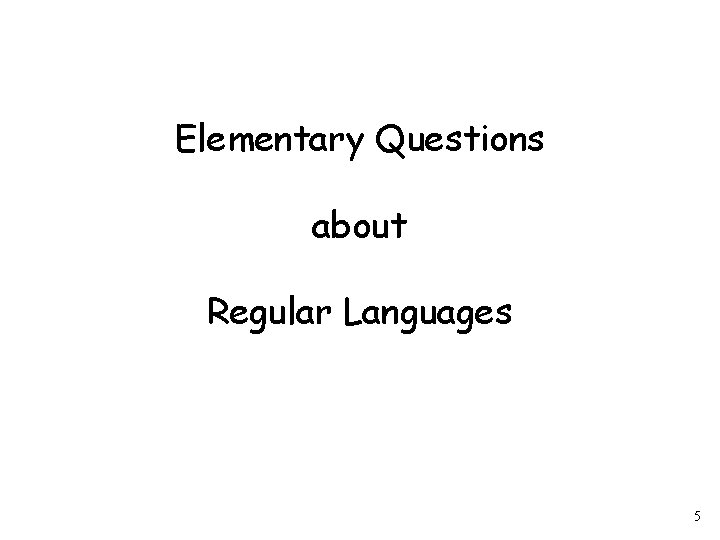Elementary Questions about Regular Languages 5Question: Given regular language how can we check if a string ? 6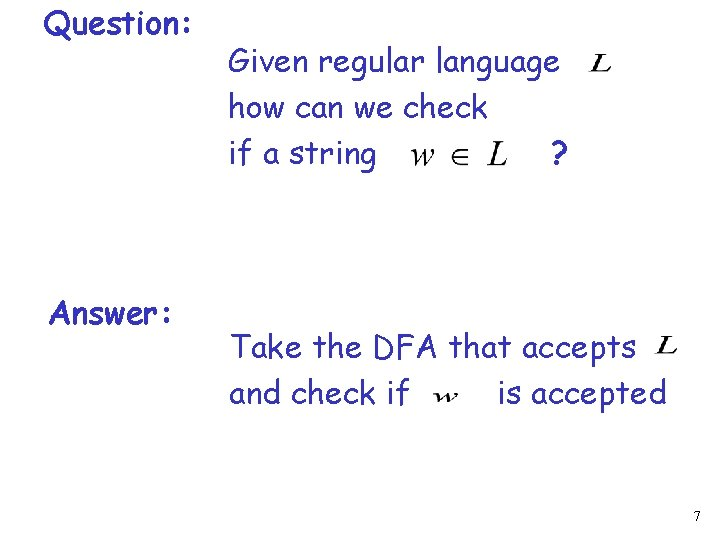Question: Answer: Given regular language how can we check if a string ? Take the DFA that accepts and check if is accepted 7Question: Answer: Given regular language how can we check if is empty, finite, infinite ? Take the DFA that accepts Then check the DFA 8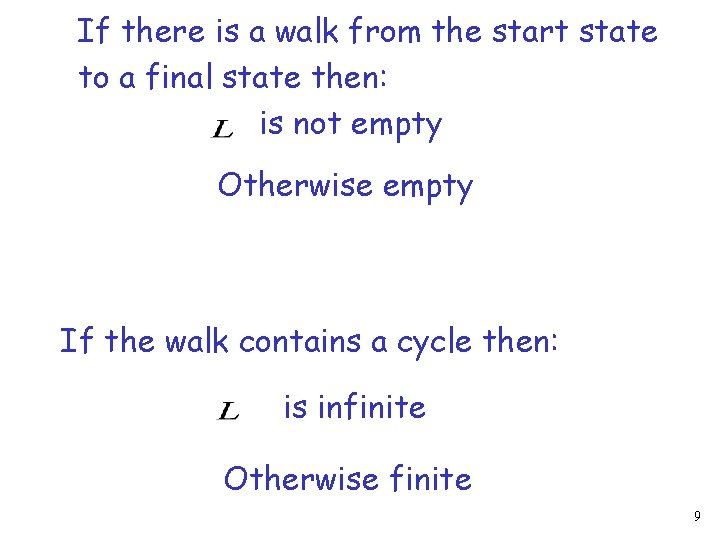If there is a walk from the start state to a final state then: is not empty Otherwise empty If the walk contains a cycle then: is infinite Otherwise finite 9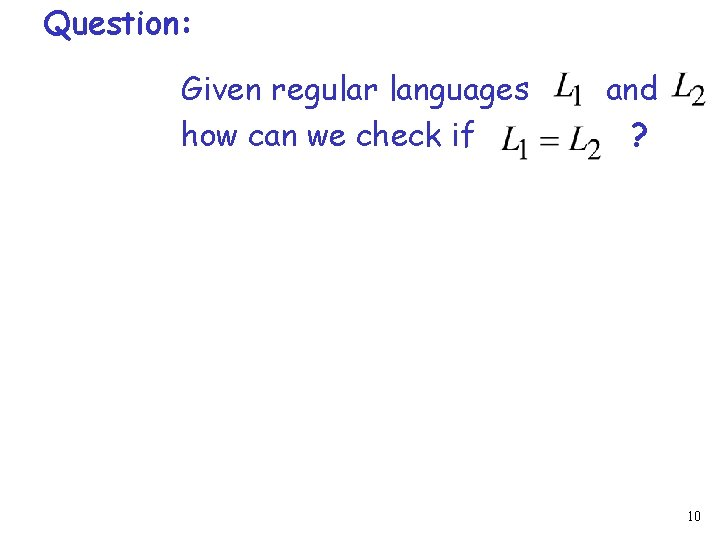Question: Given regular languages how can we check if and ? 10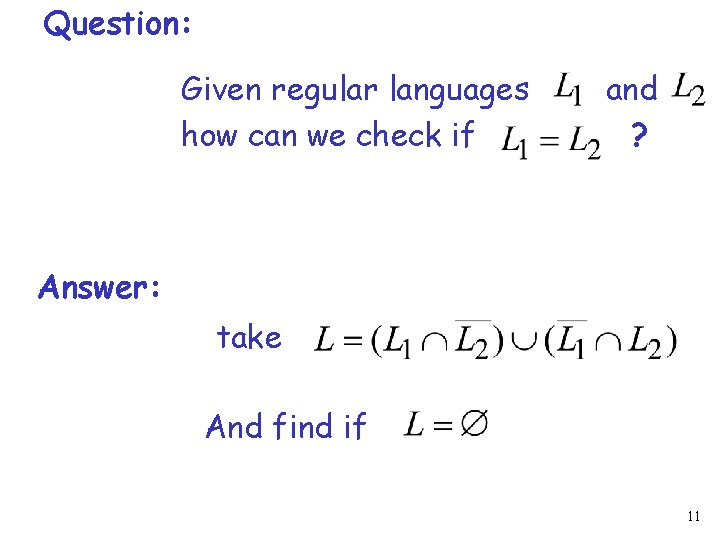Question: Given regular languages how can we check if and ? Answer: take And find if 11Question: Given language how can we check if is not a regular language ? 12Question: Given language how can we check if is not a regular language ? Answer: The answer is not obvious We need the Pumping Lemma 13The Pigeonhole Principle 14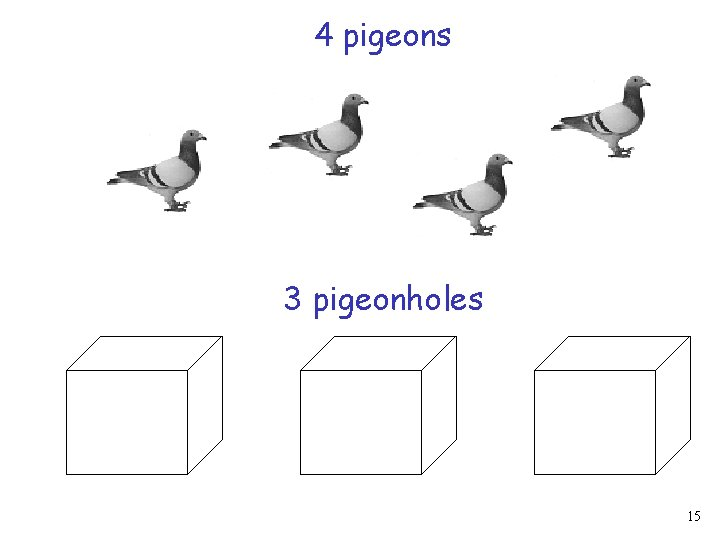4 pigeons 3 pigeonholes 15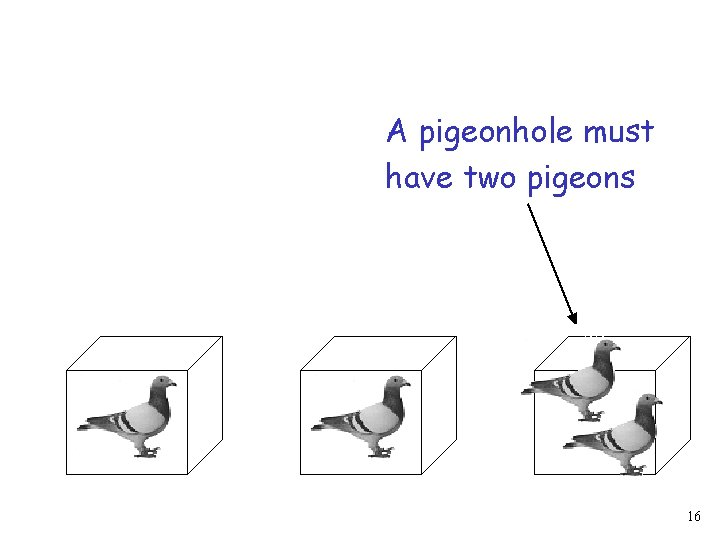A pigeonhole must have two pigeons 16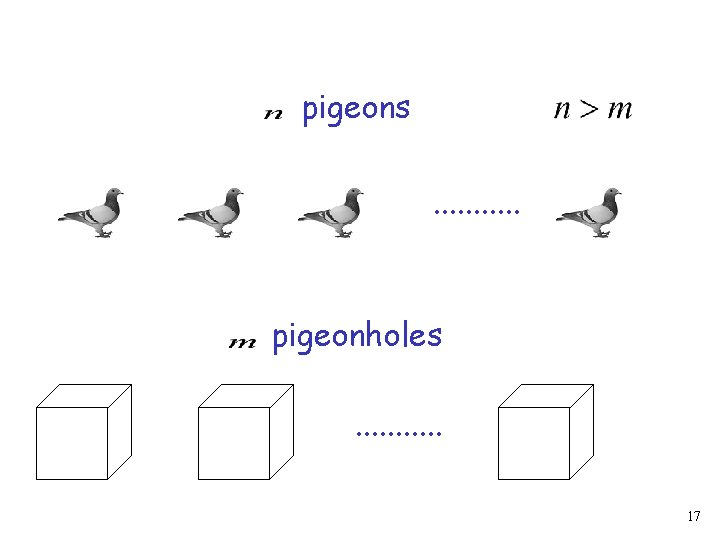pigeons. . . pigeonholes. . . 17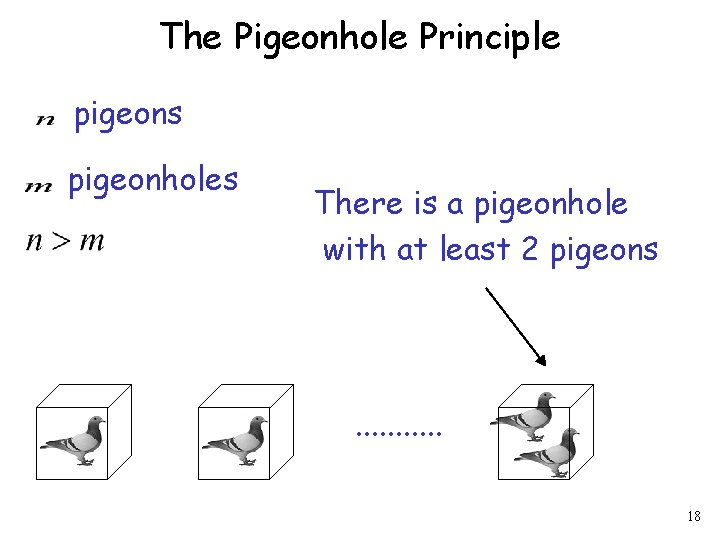The Pigeonhole Principle pigeons pigeonholes There is a pigeonhole with at least 2 pigeons . . . 18The Pigeonhole Principle and DFAs 19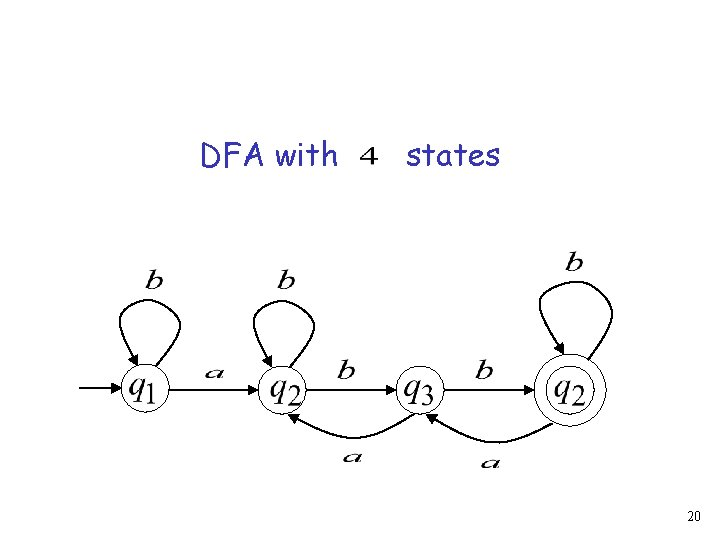DFA with states 20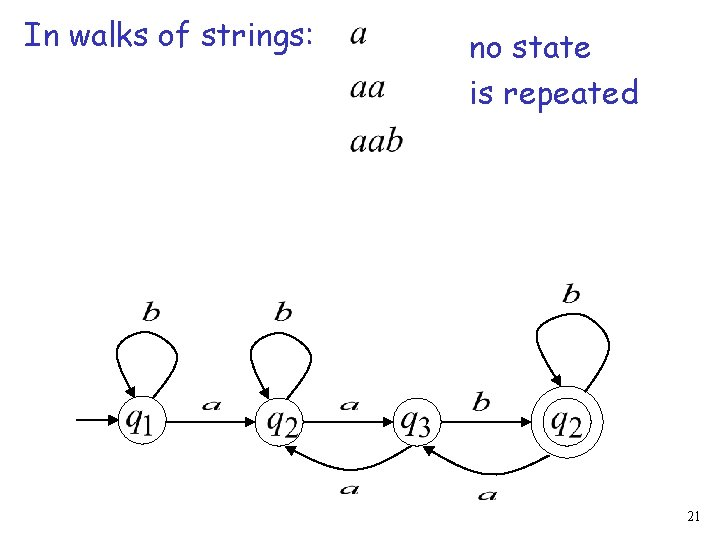In walks of strings: no state is repeated 21In walks of strings: a state is repeated 22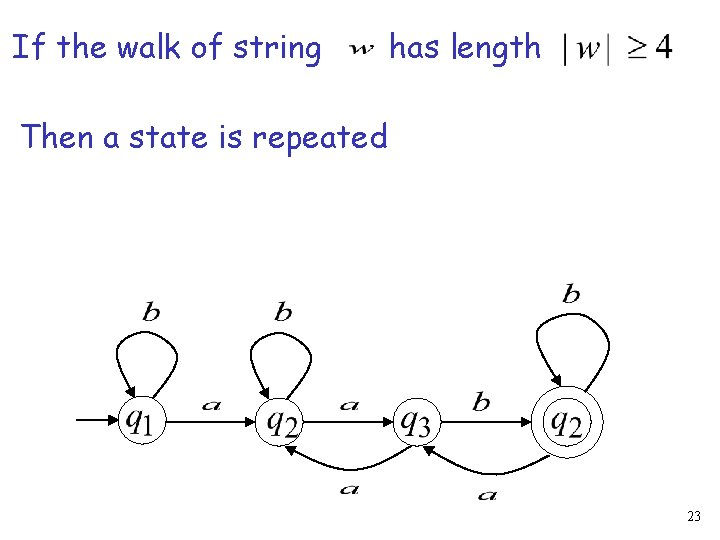If the walk of string has length Then a state is repeated 23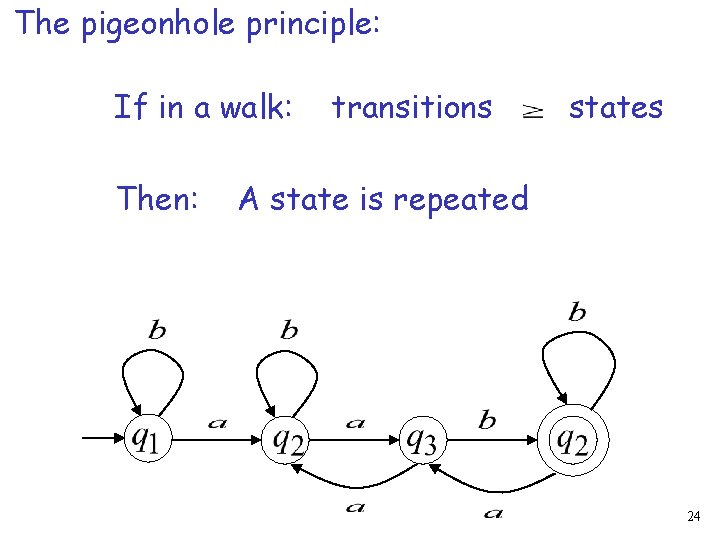The pigeonhole principle: If in a walk: Then: transitions states A state is repeated 24In other words: transitions are pigeons states are pigeonholes 25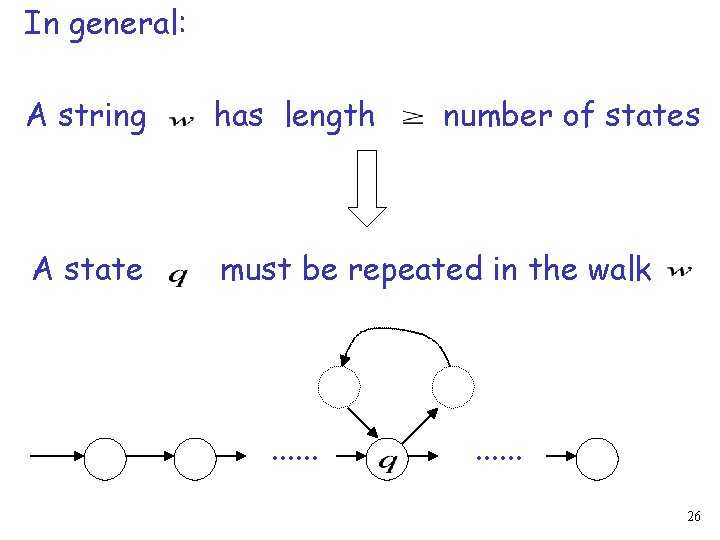In general: A string has length A state must be repeated in the walk . . . number of states . . . 26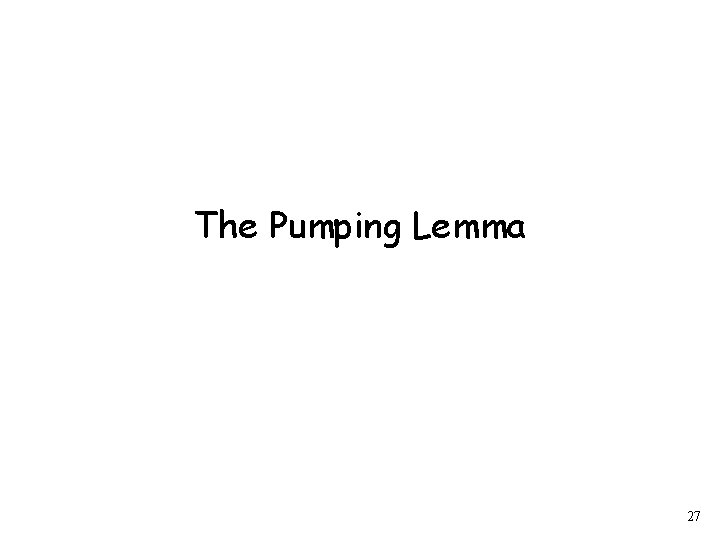The Pumping Lemma 27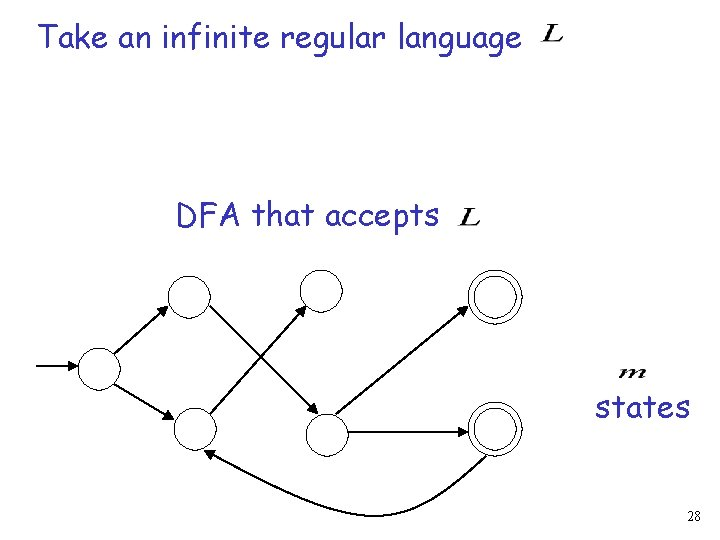Take an infinite regular language DFA that accepts states 28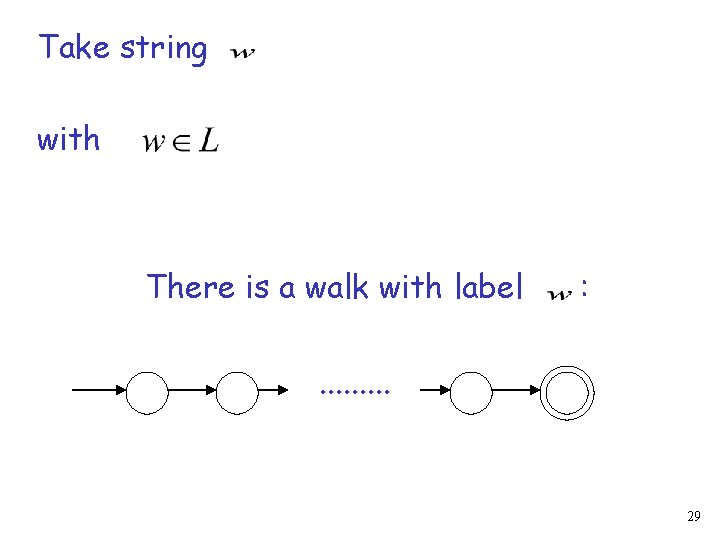Take string with There is a walk with label : . . 29If string number of states has length Then, from the pigeonhole principle: A state is repeated in the walk . . . 30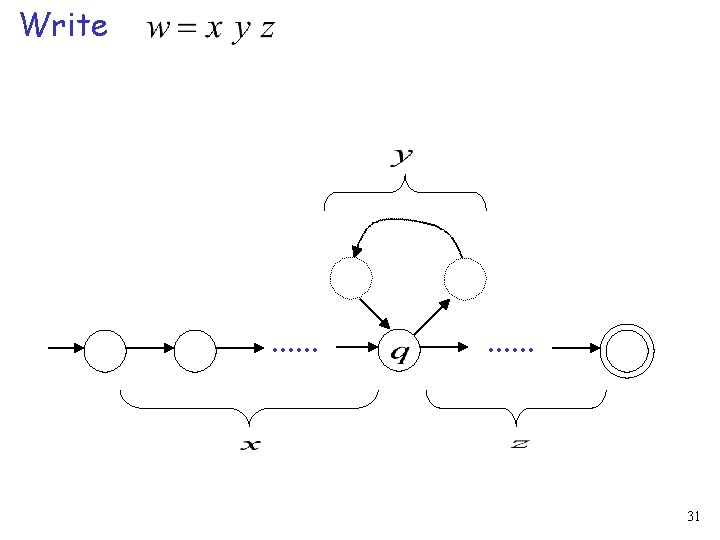Write . . . 31Observations : number of states length . . . 32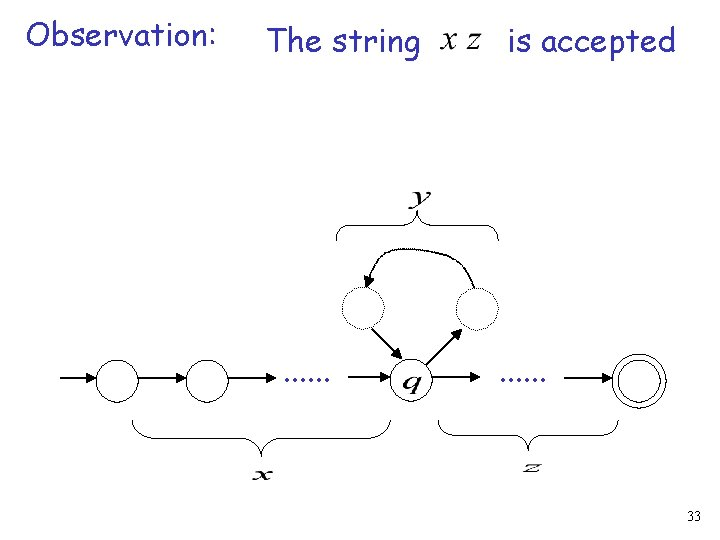Observation: The string . . . is accepted . . . 33Observation: The string is accepted . . . 34Observation: The string is accepted . . . 35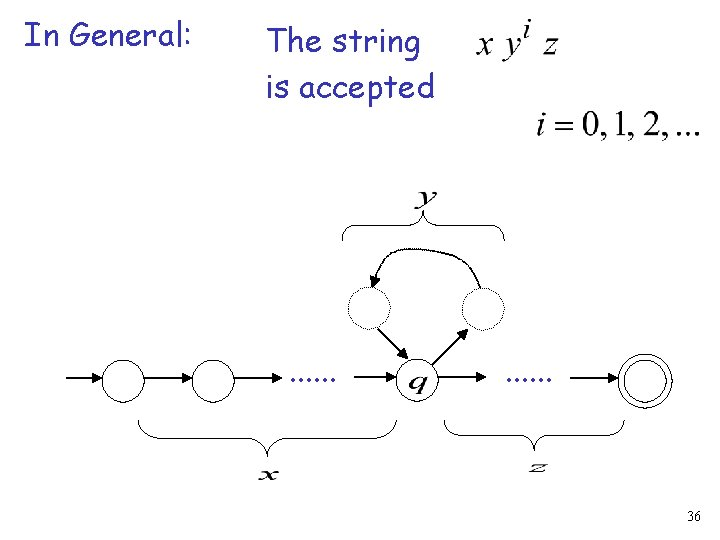In General: The string is accepted . . . 36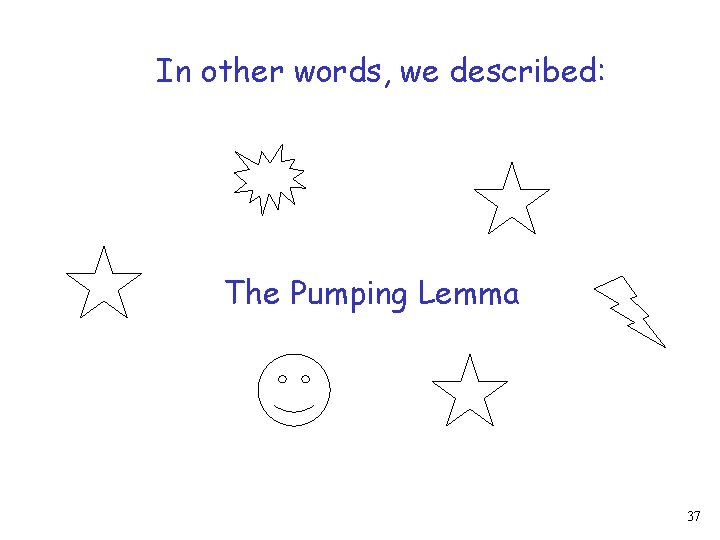In other words, we described: The Pumping Lemma 37The Pumping Lemma: 1. Given a infinite regular language 2. There exists an integer 3. For any string with length 4. We can write 5. With and 6. Such that: string 38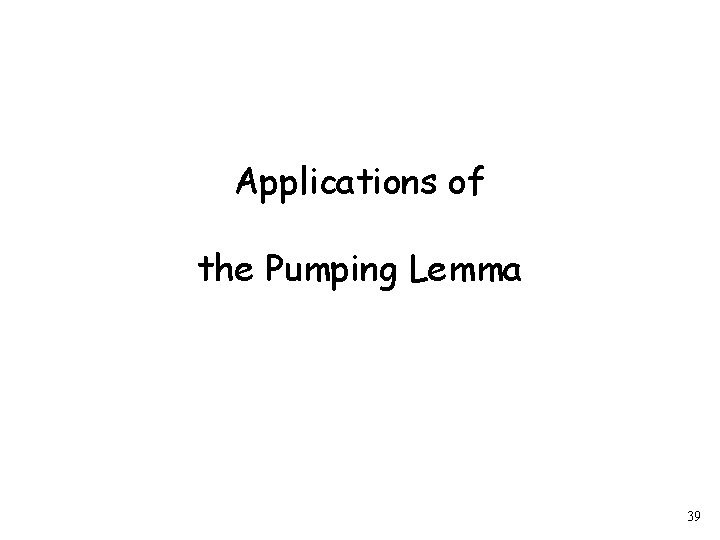Applications of the Pumping Lemma 39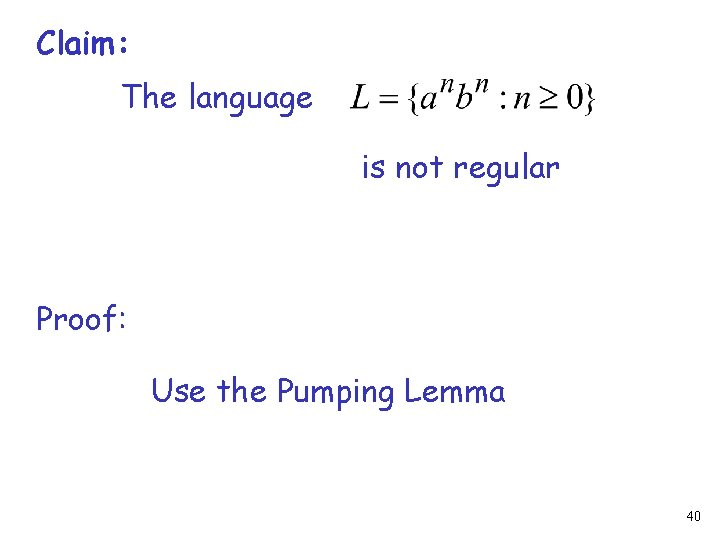Claim: The language is not regular Proof: Use the Pumping Lemma 40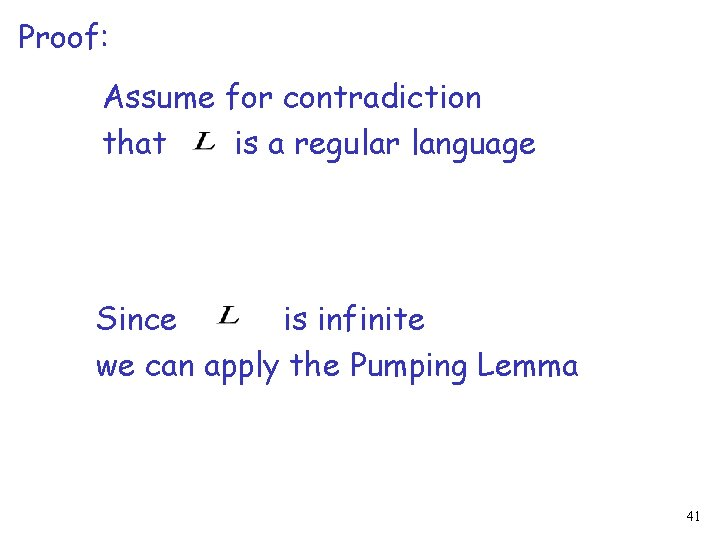Proof: Assume for contradiction that is a regular language Since is infinite we can apply the Pumping Lemma 41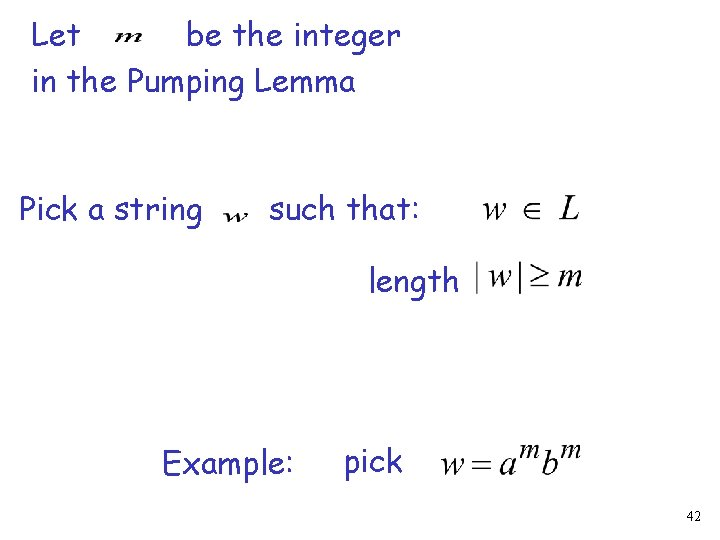Let be the integer in the Pumping Lemma Pick a string such that: length Example: pick 42Write From the Pumping Lemma it must be that length Therefore: 43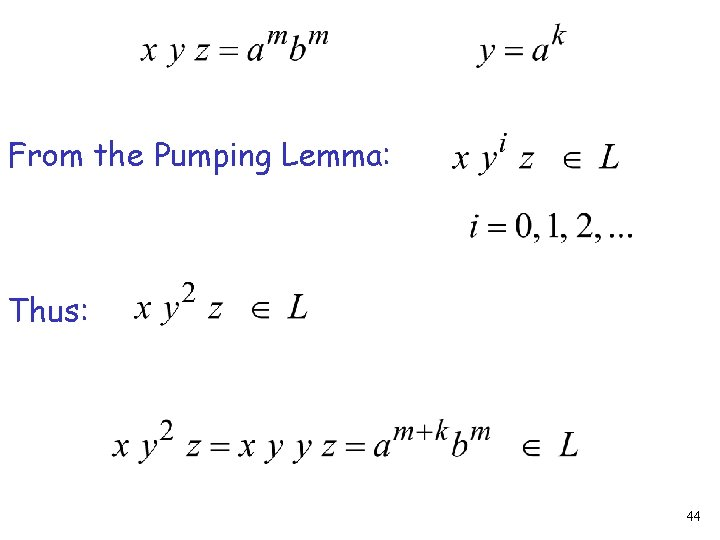From the Pumping Lemma: Thus: 44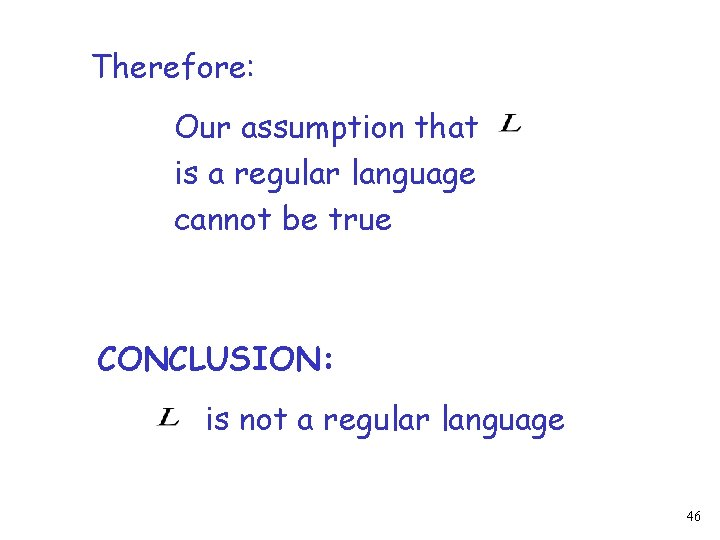Therefore: Our assumption that is a regular language cannot be true CONCLUSION: is not a regular language 46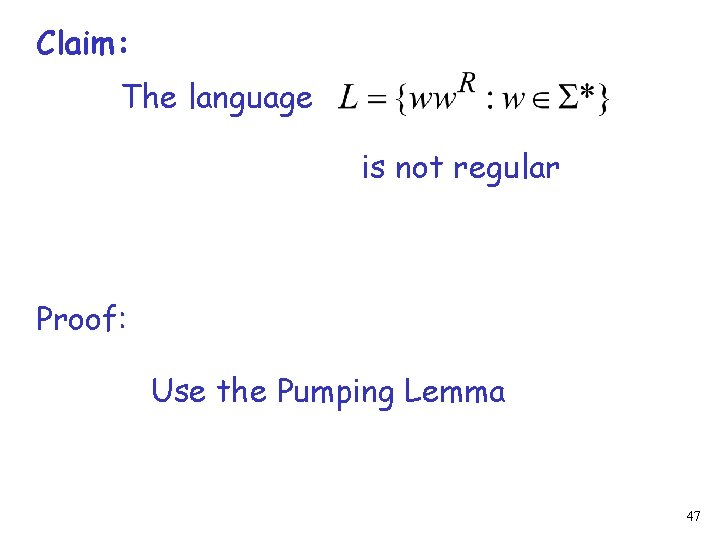Claim: The language is not regular Proof: Use the Pumping Lemma 47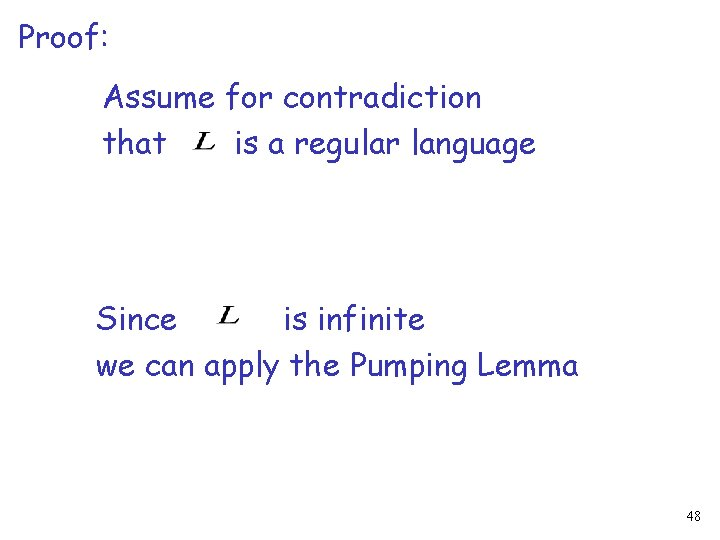Proof: Assume for contradiction that is a regular language Since is infinite we can apply the Pumping Lemma 48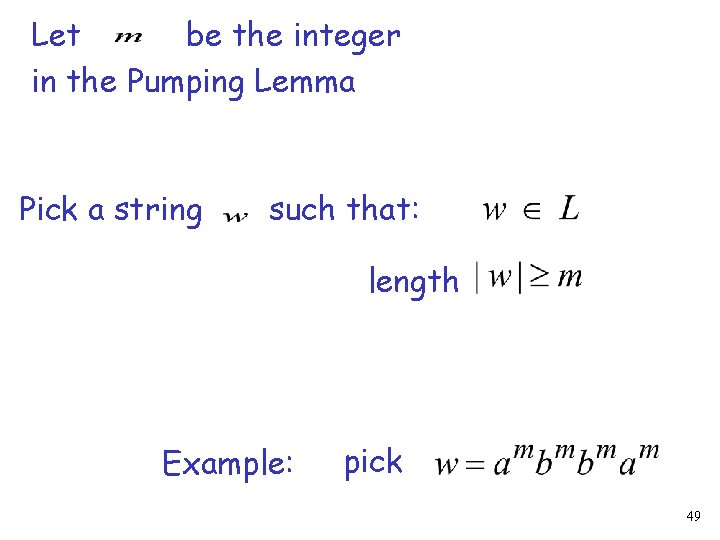Let be the integer in the Pumping Lemma Pick a string such that: length Example: pick 49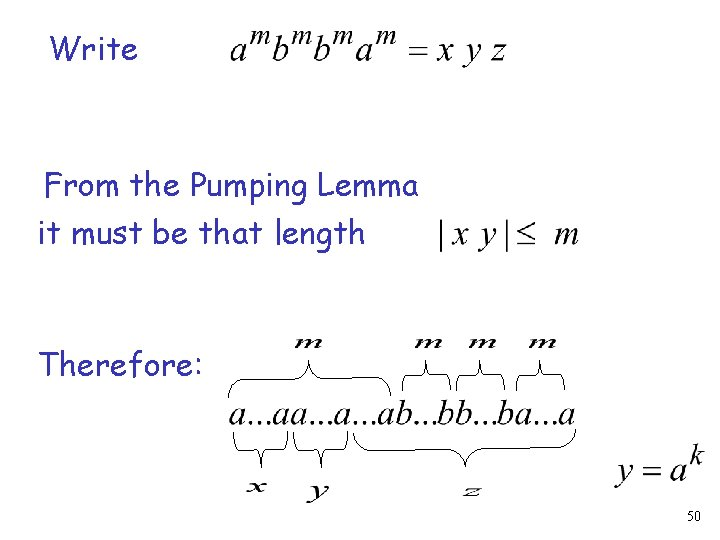Write From the Pumping Lemma it must be that length Therefore: 50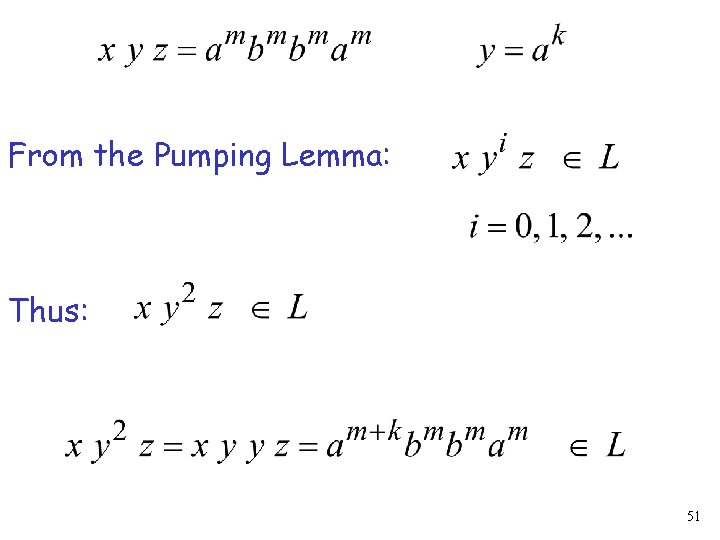From the Pumping Lemma: Thus: 51